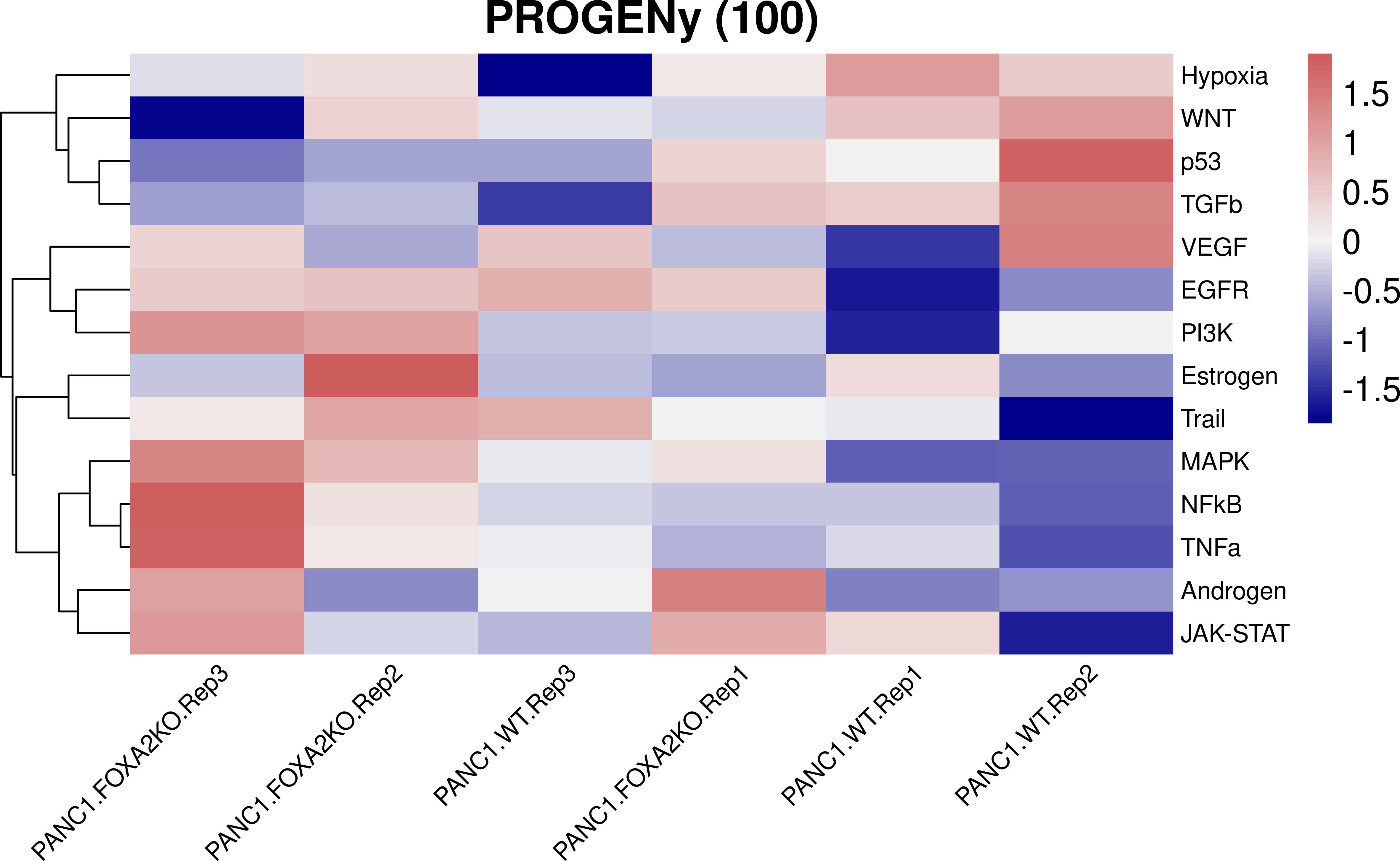# Contents

## 0.1 Introduction

This is the third part in a series of transcriptomics tutorials. We present here how to estimate pathway activity from transcriptomics data using PROGENy.

Conventional pathway analysis methods rely on the gene expression of the pathway members. However, this approach overlooks the effect of post-translational modifications and only captures very specific experimental conditions. To overcome these limitations, PROGENy (Pathway RespOnsive GENes) estimates the activity of relevant signaling pathways based on consensus gene signatures obtained from perturbation experiments (Schubert et al. 2018)

PROGENy initially contained 11 pathways and was developed for the application to human transcriptomics data. It has been recently shown that PROGENy is also applicable to mouse data and it has been expanded to 14 pathways (Holland et al., 2019). In addition, PROGENy can be applied to scRNA-seq data, as described in (Holland et al., 2020)

PROGENy is available as a Bioconductor package. For additional information about the PROGENy method, visit its website:

https://saezlab.github.io/progeny/

## 0.2 Getting Started

We first load the required libraries.

``````library(progeny)
library(tibble)
library(tidyr)
library(dplyr)
library(pheatmap)
library(ggplot2)``````

### 0.2.1 Import the raw count dataframe

The data can be downloaded from: https://www.ncbi.nlm.nih.gov/geo/query/acc.cgi?acc=GSE119931 The file name is: GSE119931_PANC1.FOXA2KO.genes.counts.txt.gz

``````## We assign the normalised counts and the experimental design
data("vignette_data")

Normalised_counts <- vignette_data\$counts
Experimental_design <- vignette_data\$design

## We read the results from the differential analysis.
ttop_KOvsWT <- vignette_data\$limma_ttop``````

We have to slightly modify the format of the input files to make it suitable for running Progeny.

``````Normalised_counts_matrix <- Normalised_counts %>%
dplyr::mutate_if(~ any(is.na(.x)),~ if_else(is.na(.x),0,.x)) %>%
tibble::column_to_rownames(var = "gene") %>%
as.matrix()

ttop_KOvsWT_matrix <- ttop_KOvsWT %>%
dplyr::select(ID, t) %>%
dplyr::filter(!is.na(t)) %>%
column_to_rownames(var = "ID") %>%
as.matrix()``````

## 0.3 Pathway activity with Progeny

We first compute Progeny scores for every sample (with the replicates) using the normalised counts. It is worth noting that we are going to use the 100 most responsive genes per pathway. This number can be increased depending on the coverage of your experiments. For instance, the number of quantified genes for single-cell RNA-seq is smaller than for Bulk RNA-seq or microarray. In those cases, we suggest to increase the number of responsive genes to 200-500.

``````PathwayActivity_counts <- progeny(Normalised_counts_matrix, scale=TRUE,
organism="Human", top = 100)
Activity_counts <- as.vector(PathwayActivity_counts)``````

We present the results in a heatmap:

``````paletteLength <- 100
myColor <-
colorRampPalette(c("darkblue", "whitesmoke","indianred"))(paletteLength)

progenyBreaks <- c(seq(min(Activity_counts), 0,
length.out=ceiling(paletteLength/2) + 1),
seq(max(Activity_counts)/paletteLength,
max(Activity_counts),
length.out=floor(paletteLength/2)))

progeny_hmap <- pheatmap(t(PathwayActivity_counts),fontsize=14,
fontsize_row = 10, fontsize_col = 10,
color=myColor, breaks = progenyBreaks,
main = "PROGENy (100)", angle_col = 45,
treeheight_col = 0,  border_color = NA)``````Now, we run an enrichment analysis using a competitive permutation approach to assess the significance of the pathway activity. We end up with Normalised Enrichment Scores (NES) for each pathway.

``````PathwayActivity_zscore <- progeny(ttop_KOvsWT_matrix,
scale=TRUE, organism="Human", top = 100, perm = 1000, z_scores = TRUE) %>%
t()
colnames(PathwayActivity_zscore) <- "NES"``````
``````PathwayActivity_zscore_df <- as.data.frame(PathwayActivity_zscore) %>%
rownames_to_column(var = "Pathway") %>%
dplyr::arrange(NES) %>%
dplyr::mutate(Pathway = factor(Pathway))

ggplot(PathwayActivity_zscore_df,aes(x = reorder(Pathway, NES), y = NES)) +
geom_bar(aes(fill = NES), stat = "identity") +
scale_fill_gradient2(low = "darkblue", high = "indianred",
mid = "whitesmoke", midpoint = 0) +
theme_minimal() +
theme(axis.title = element_text(face = "bold", size = 12),
axis.text.x =
element_text(angle = 45, hjust = 1, size =10, face= "bold"),
axis.text.y = element_text(size =10, face= "bold"),
panel.grid.major = element_blank(),
panel.grid.minor = element_blank()) +
xlab("Pathways")``````